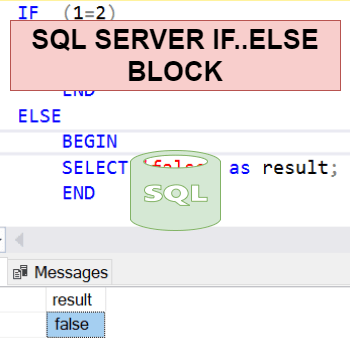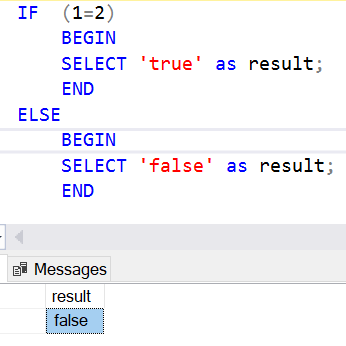Home » SQL SERVER IF..ELSE

# SQL SERVER IF..ELSESQL Server IF..ELSE block is a control-of-flow that allows you to execute or skip a statement block based on a specified condition.

SYNTAX

```IF Boolean_expression
{ sql_statement | statement_block }
[ ELSE { sql_statement | statement_block } ]```

Boolean_expression
Is an expression that returns TRUE or FALSE.

IF boolean expression evaluates to TRUE then the sql_statement | statement_block is executed. Otherwise, the sql_statement | statement_block is skipped and the control of the program is passed to the ELSE  statement. ELSE is an optional.

If the Boolean expression using a SELECT statement then SELECT statement must be enclosed in parentheses.

{ sql_statement| statement_block }
It uses the control-of-flow keywords BEGIN and END.

Lets look at an example of IF..ELSE block in SQL Server.

IF..ELSE BLOCK

Following statement evaluates an expression in IF statement and if it is evaluated as TRUE then the statement block in IF statement is executed otherwise it executes the statement block inside ELSE block.

As you can see, an expression that is evaluated by if statement that is 1=2 , obviously it returns false as 1 can not be equal to 2, so the control is passed to ELSE block and execute the statement. So, it returns the ‘false’.

```IF (1=2)
BEGIN
SELECT 'true' as result;
END
ELSE
BEGIN
SELECT 'false' as result;
END```Lets change the expression 1=1 as it is TRUE , now IF statement will returns TRUE and execute the statement inside IF block and returns ‘TRUE’

```IF (1=1)
BEGIN
SELECT 'true' as result;
END
ELSE
BEGIN
SELECT 'false' as result;
END```NESTED IF..ELSE BLOCK

Following statement uses nested IF..ELSE statement within inside another IF..ELSE  statement.

We have declared a variable @val and assigned value =5 to it.

Now IF statement checks for given condition that is 1=1, which is true so it enters in IF statement block where it has another IF statement block that is called as NESTED IF..ELSE block, In this IF statement block condition @val=3 is checked which is 5=3 that is evaluated as FALSE so control is passed to ELSE block of Nested IF.. ELSE statement.

Now statement in ELSE block is executed and it returns  NESTED IF_ELSE result is FALSE

```Declare @val int =5

IF (1=1)
BEGIN
IF (@val=3)
BEGIN
SELECT 'NESTED IF_ELSE result is TRUE' as result;
END
ELSE
BEGIN
SELECT 'NESTED IF_ELSE result is FALSE' as result;
END
END
ELSE
BEGIN
SELECT 'false' as result;
END```